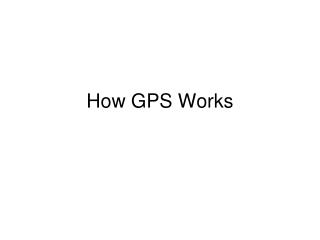Download PresentationHow GPS Works

# How GPS Works - PowerPoint PPT PresentationDownload Presentation## How GPS Works

- - - - - - - - - - - - - - - - - - - - - - - - - - - E N D - - - - - - - - - - - - - - - - - - - - - - - - - - -
##### Presentation Transcript

1. How GPS Works

2. NAVSTAR • NAVigation Satellite Timing And Ranging • Operated by Air Force • Launch Weight 1800 kg (4000 lb) • Orbital radius 20,200 km • Orbital Period 12 hours • Inclination 55 degrees • 24 active, 4 spare • 4 planes, 6 satellites per plane

3. Locating Earthquakes

4. Locating Earthquakes - Depth

5. GPS and Seismology • GPS is a lot like locating earthquakes • Use Trilateration • Locate an unknown point using distances from three known points • Unknown point is actually at intersection of three spheres (in earth, sort of) • One important difference • Earthquakes create signals with different speeds so distance is coded in the signal

6. The Timing Problem • Travel time = Radius to satellite • Distance to NAVSTAR: 14,000-20,000 km • Travel time =.05-.07 seconds • Have to measure travel time to sub-ppm accuracy • How do you know radius if you don’t know where you are? • Synchronized signals in GPS unit and satellite

7. Signals in GPS

8. An Early Pseudo-Random Code • 1776 (seed) • 17762 = 3154176 • 15412 = 2374681 • 37462 = 14032516 • 40322 = 16257024 • 62572 = 39150049 • 91502 = 83722500, etc.

9. The Clock Problem • Atomic clocks costs thousands of dollars • In the overall budget of a satellite program, that’s chump change • In the cost of a hand-held GPS unit, not so much • GPS units use inexpensive quartz clocks • Not precise enough • One microsecond = 300 meters • Have satellites broadcast time signals? • How do we know the travel time? • Unpredictable timing error introduced

10. Timing Errors • Satellite positions known precisely and ephemeris (or almanacs) updated regularly • If distances r1, r2, r3 are known, we can solve for latitude, longitude, and elevation • 3 variables, 3 unknowns • If we also have to solve for an unknown timing error, three radii are insufficient

11. Effect of Timing Error

12. Correcting Clock Errors • We have four unknowns: latitude, longitude, elevation and error • Can solve with four satellite fixes • Once error is known, can reset GPS unit clock • This is why the magic number of four for GPS signals • Full x,y,z solution = 3D

13. What About Less Than Four? • We have four unknowns: latitude, longitude, elevation and error • Three satellites aren’t enough • But we have a fourth sphere: the Earth • May have to make assumptions about radius due to oblateness of earth and topography • Can get latitude and longitude (2D) and accuracy limited